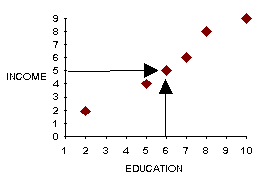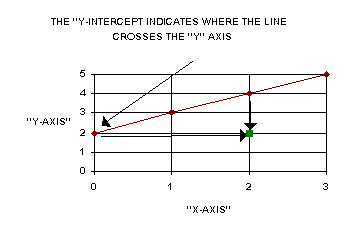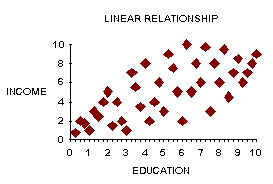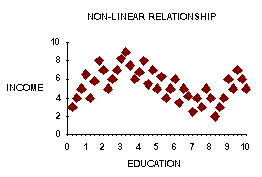previous lesson

Prediction

INTERVAL DATA -- Bivariate Distribution

A. A review of predictive techniques:
 Income (Y) Education (X) Hi Lo Hi 14 2 Lo 6 11

Here one can easily see a positive relationship:

hi education--hi income

low education--low income

It is easy to predict using this table: Say a person has a low income, one would predict a low level of education.

Data in different form:
 Person Education X Income Y A 10 9 B 8 8 C 7 6 PREDICT 6 5 D 5 4 E 2 2

Using this information: If a person has education 8, predict an income of 8. If a person has income 5, although there is no income of 5, still predict that the person's education would be about 6.

A scatter diagram can be made to represent the relationship between the two variables: X = Education, Y = Income.A straight line approximately describes the relationship between X and Y. One can use it to predict: Say a person has an income of 5, one would predict an education of about 6.

B. Equation of a Line: Y = a + bX

Where: X and Y are variables using for prediction

a = "Y - intercept"

b = slope of lineTo get the slope, take any point not on the line and measure its distance from the line. The vertical line's distance is "P" and the horizontal line's distance is "Q." The slope equals P / Q. In this example,

a = 2, b = 2 / 2 = 1, so the equation of this line is:

Y = 2 + 1X

C. Predicting Y from a knowledge of X: Y = ayx + byxX

Where: byx = N( XY) - ( X)( Y)
----------------N( X2 ) - (X)2

ayx = Y - (byx)(X)
------------N

D. Predicting X from a knowledge of Y: X = axy + bxyY

Where: byx = N( XY) - ( X)( Y)
----------------N(Y2 ) - ( Y)2

ayx = X - (byx )(Y)
-----------N

Assumptions:

interval data

linear relationship

homoscedasticityThere is a linear relationship as long as the shape of the data scatter has some oblong shape. A circle, a S, etc. is not a linear. Homoscedasticity: similar variance in columns and rows. If it fits linear a relationship, it will also be homoscedastic.

Example: Q: Predict the income of a person who is 9 feet tall.

Before using the formulas, try predicting by observation; one would expect an answer of about \$9.50.
 Person Height X Income Y A 10 10 B 8 9 C 6 7 D 3 2

A: X = height Y = income
 Person Height X X2 Income Y XY A 10 100 10 100 B 8 64 9 72 C 6 36 7 42 D 3 9 2 6 N=4 27 209 28 220

byx = [4(220) - 27(28)] / [4(209) - (27)2] = 1.16

ayx = [28 - 1.16(27)] / 4 = -.83

Y = -.83 + 1.16X

This is the straight line which describes the data. Now use the equation to solve for X = 9:
Y = -.83 + 1.16(9)
Y = -.83 + 10.44
Y = \$9.61